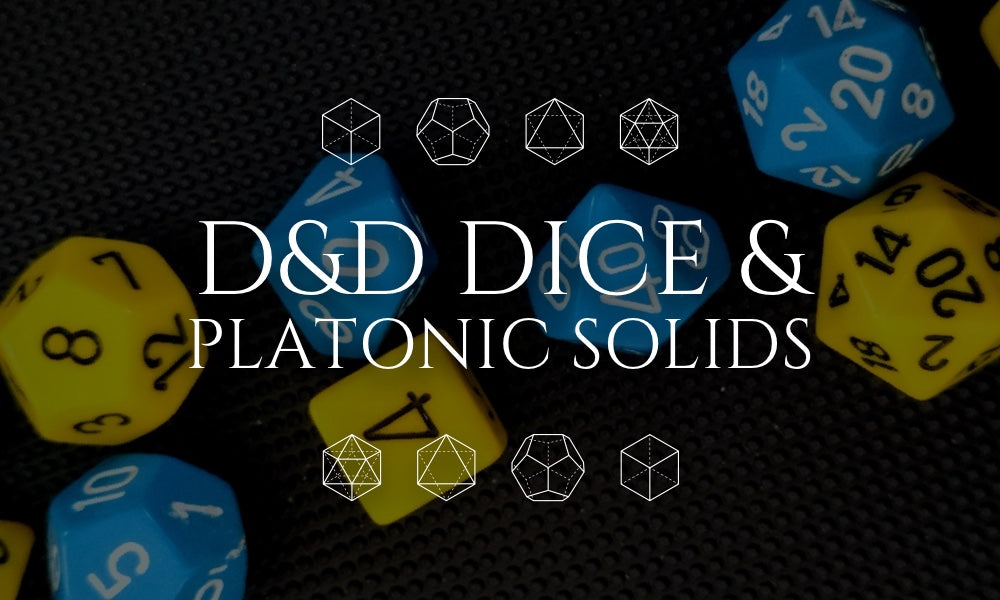# D&D Dice and Platonic Solids

Polyhedral dice, also known as role-playing dice or RPG dice. These dice are commonly used in tabletop games such as Dungeons and Dragons, and come in a variety of shapes and sizes.

One of the most important aspects of polyhedral dice is their relationship to Platonic solids. A Platonic solid is a three-dimensional shape made up of congruent, regular polygons. There are five Platonic solids: the tetrahedron (four triangular faces), hexahedron (six square faces), octahedron (eight triangular faces), dodecahedron (twelve pentagonal faces), and icosahedron (twenty triangular faces).

The tetrahedron is the simplest Platonic solid and is often used as a four-sided die, also known as a "d4". The hexahedron, or cube, is the most common polyhedral die and is used as a six-sided die, also known as a "d6". The octahedron is typically used as an eight-sided die, also known as a "d8", the dodecahedron as a twelve-sided die, also known as a "d12" and the icosahedron as a twenty-sided die, also known as a "d20".

The mathematics of these dice is related to the geometry of the Platonic solids. For example, the probability of rolling a certain number on a d4 is the same as the probability of landing on a certain face of a tetrahedron, and the probability of rolling a certain number on a d6 is the same as the probability of landing on a certain face of a cube.

In addition to the traditional Platonic solids, there are also other types of polyhedral dice that are not based on Platonic solids. For example, the d10 is a die that has ten faces and is typically used for percentile rolls. It does not have a corresponding Platonic solid, but it still follows the same principles of probability as the other dice.

Overall, polyhedral dice are an important aspect of tabletop gaming and are closely related to the mathematics of Platonic solids. Understanding the properties of these solids can help players make more informed decisions in their games, and can also add an extra layer of complexity to the game. It's also worth mentioning that many less common types of polyhedral dice exist, and some of them are not based on Platonic Solids, for example, there are d3, d5, d7 and d14.

Polyhedral dice are an important tool in tabletop gaming and their relation to Platonic solids gives them a unique mathematical structure. Understanding the probabilities and geometry of these shapes can add an extra layer of strategy to gameplay and make for a more engaging experience.